## Nov 15, 2010

### Color-coded contour lines with Mathematica

In ContourPlot, ContourStyle –> function can be used to color-code contour lines.For example, the number of contours is numC=20, then for each contour, the color is defined by a coloring function:

ContourStyle -> Table[{ColorData["Rainbow", (i - 1)/(numC - 1)]}, {i, numC}]

Of course, it is also necessary to set Contours –> numC.

Here are the examples, the famous peaks function from Matlab is used.

2D ContourPlot example: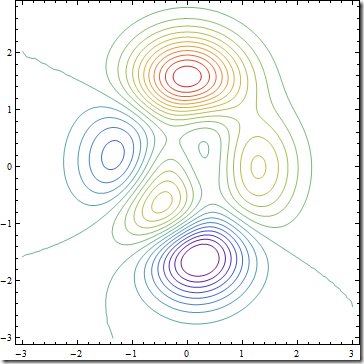Turn 2D plot into the 3D one:Another 3D example: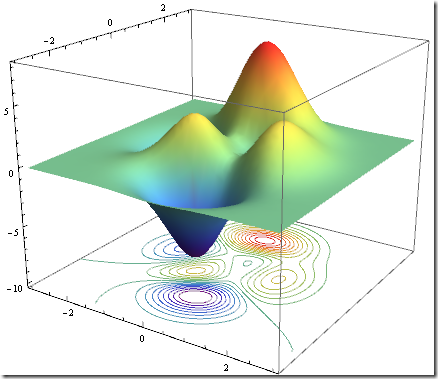Download the notebook.

## Nov 8, 2010

### Customizing DateListPlot with PlotMarkers

This example shows you how to specific PlotMarkers for each point in a data set. The solution is quite simple, just partition the dataset into different series, each set only contains one point exactly: Partition[data, 1]. Then we can assign the different PlotMarkers for each point.

Let’s download some weather data:

data = WeatherData[\$GeoLocation, "WindDirection", {{2009, 1, 1}, {2009, 1, 5}}];
data = Select[data, FreeQ[#, {_, Missing["NotAvailable"]}] &];

We like to use arrows to represent wind directions:

markers =
Graphics[{Red, Arrow[{{0, 0}, -0.5 {Sin[#[] Degree], Cos[#[] Degree]}}],
EdgeForm[], FaceForm[], Rectangle[{-1, -1}, {1, 1}]}] & /@ data;

We put a invisible box around arrow to make sure that the markers are aligned by {0, 0}.

newdata = data; newdata[[All, 2]] = 0;
g = DateListPlot[Partition[newdata, 1], PlotRange -> All, PlotMarkers -> markers, Axes -> {True, False}, FrameTicks -> {Automatic, None}]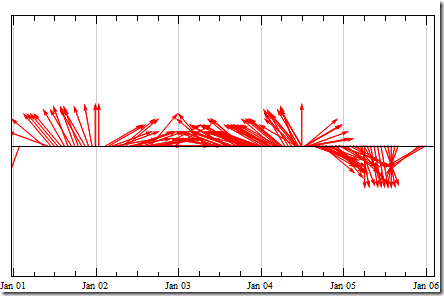Let’s test another place: Tokyo, Japan

Summer time: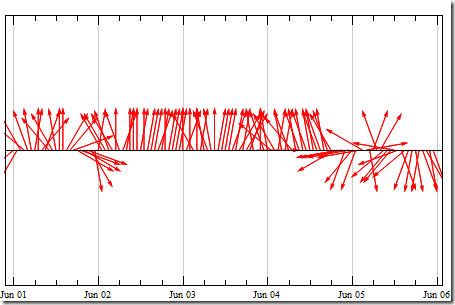Winter time:The pattern of winter “north” wind and the summer “south” wind is very clear, it is much better than just plotting points.

No notebook, all the codes are here already.

## Nov 1, 2010

### Plotting on Google static map

We have given an example on plotting with WMS. You may be wondering how we can do the similar thing with Google static map. It is very easy to use with Mathematica:

googleMap[{lat_,lon_},{sizex_,sizey_},zoom_,maptype_]:=Import["http://maps.google.com/maps/api/staticmap?sensor=false&center="<>ToString[lat]<>","<>ToString[lon]<>"&zoom="<>ToString[zoom]<>"&size="<>ToString[sizex]<>"x"<>ToString[sizey]<>"&maptype="<>maptype];

Google map is based on Mercator Projection. So the data has to be projected, rather than drawing (longitude, latitude) pairs directly. The scale on east-west (longitude) is constant with zoom level, and only latitude needs to be projected.

(* convert lat to y *)
lat2y[lat_]:=0.5Log[(1+Sin[lat Degree])/(1-Sin[lat Degree])];
(* convert y to lat *)
y2lat[y_]:=Gudermannian[y]*180/Pi;

We have the data in pairs of (longitude, latitude) which lie in a bounding box ((lon1,lat1), (lon2, lat2)), and to plot them on a Google static map, we need: 1. find the center of the map; 2. find the right zoom level; 3. find the proper map size; 4. project the data: (longitude, lat2y[latitude])

I will skip the details, if you are interested into the mathematics, check out these two posts, : R-Google Map and How to make Google static maps interactive.

Here are two examples:

coords = CountryData["Australia", "Coordinates"];
(* only project latitude *)
Map[{#[],lat2y[#[]]}&, coords,{2}]]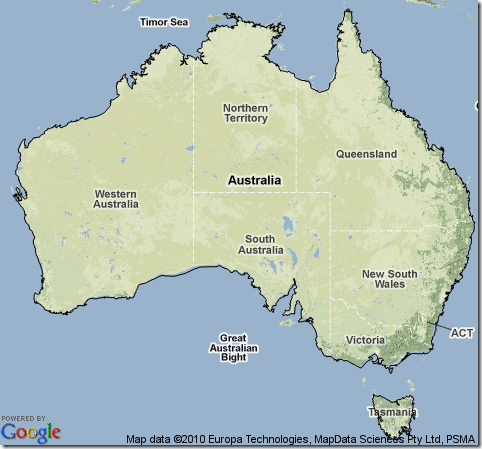In the second example, GIS data is imported from an XML file, it is from the post before Mathematica 7.0 released.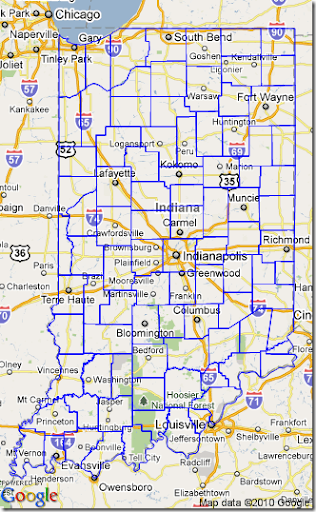Mathematica 8 has the texture function, so we can import static Google map as the texture, it will be easy to create complex 3D GIS visualization.

Download the notebook for detail.